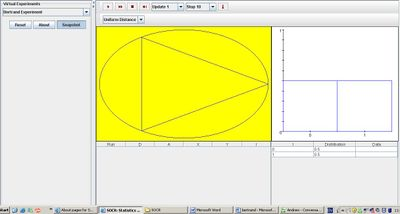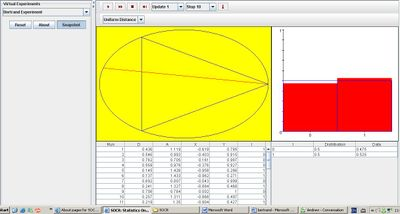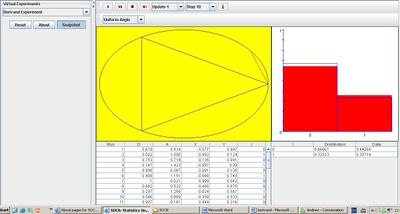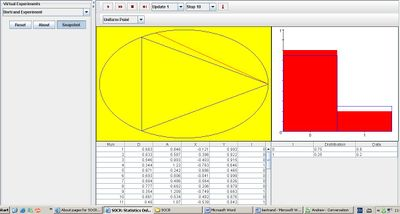# SOCR EduMaterials Activities BertrandExperiment

## Description

The Bertrand’s experiment generates a random chord of a circle with one point fixed at (1,0).

In the table, a random point (X,Y) is recorded on each update as well as D, the length of the line segment from the center of the circle to the center of the chord, and A, the angle that this line segment makes with the horizontal. Variable I indicates the event that the chord is longer than the length of a side of the inscribed equilateral triangle with 0 as shorter than the length of a side and 1 as longer than the length of a side. The density and empirical density of I is recorded in the distribution table.

On each update, the density of I is shown in blue and the empirical density of I is shown in red in the distribution graph.

Three different models can be selected with the list box: 1. The model where the distance D is uniformly distributed 2. The model where angle A is uniformly distributed 3. The model where the coordinate X is uniformly distributed

## Goal

To provide a simple generator that displays different models that satisfy the geometric properties of a Bertrand experiment.

## Experiments

Go to the SOCR Experiment [] and select the Bertrand’s Experiment from the drop-down list of experiments on the top left. The image below shows the initial view of this experiment:When pressing the play button, one trial will be executed and recorded in the distribution table below. The fast forward button symbolizes the nth number of trials to be executed each time. The stop button ceases any activity and is helpful when the experimenter chooses “continuous,” indicating an infinite number of events. The fourth button will reset the entire experiment, deleting all previous information and data collected. The “update” scroll indicates nth number of trials (1, 10, 100, or 1000) performed when selecting the fast forward button and the “stop” scroll indicates the maximum number of trials in the experiment.

Uniform Distance

When “Uniform Distance” is selected from the list box, the density of I graph is symmetric. As the number of trials increase, the empirical density of I eventually takes the same form of the density graph. The image below demonstrates this outcome:Uniform Angle When “Uniform Angle” is selected from the list box, the density of I graph is not symmetric—the density value of 0 is larger than that of 1. Similarly, as the number of trials increase, the empirical density of I eventually takes the same form of the density graph. The image below demonstrates this outcome:Uniform Point When “Uniform Point” is selected from the list box, the density graph shown in blue displays a much larger value for 0, thus creating a very small value for 1. This shows that the probability of a chord exceeding the length of one side of the inscribed triangle is very small. As the number of trials increase, the empirical density of I eventually takes the same form of the density graph. The image below demonstrates this outcome:## Applications

The Bertrand Experiment is an example of statistics and probability involving geometry. When selecting an experiment from the perspective of uniform point, angle, or distance, the density of variable I vary in respect.

Architectures and engineers may want to use this applet when determining the density of a randomly sampled length of material for a given sample of area. Suppose a team was given the estimated length of a rectangular room that is to be remodeled and they must find the right size length of metal to outline this chosen area. By using this java applet, the members may determine the probability of randomly selecting the interval of sizes that will fit into this room.

When using a mannequin to create new types of clothing, the size of material is usually bigger than necessary. Suppose a fashion designer is managing his budgets and wants to determine the probability of choosing the right size of material for the mannequin. After running the Bertrand Experiment many times, he concludes that randomly cutting up the material will make him lose money because the material may be too small for the mannequin, thus wasting money and material. Whereas the material will always be put to use even if it is too big.

A group of students are designing a car for their mechanics class and want to know the probability of randomly selecting a small fan for the air conditioner according to its radius.